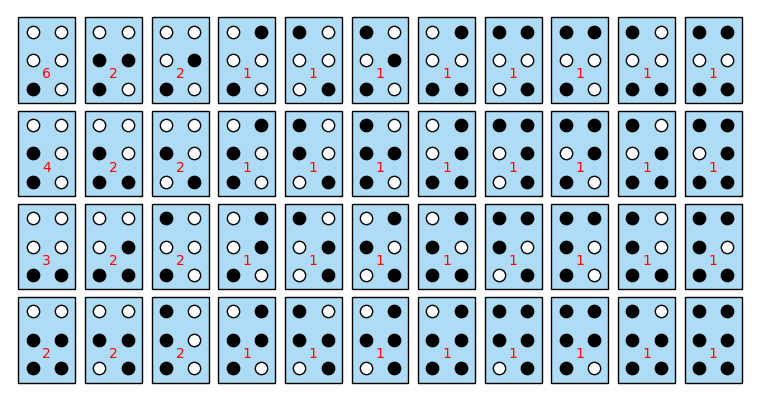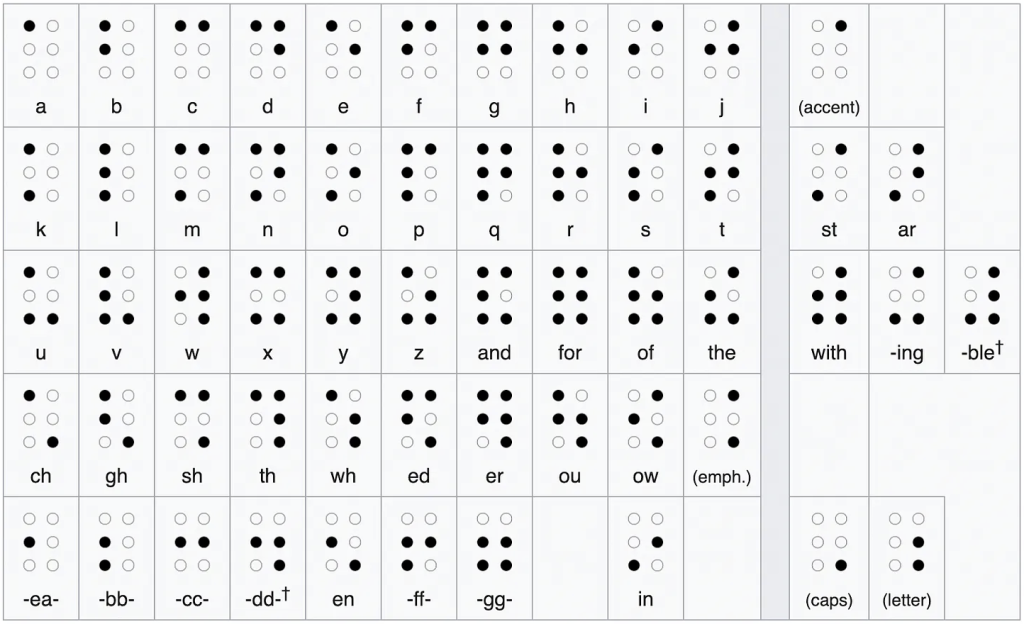# Braille puzzleThis week’s Fiddler is a counting problem about the Braille system.

Braille characters are formed by raised dots arranged in a braille cell, a three-by-two array. With six locations for dots, each of which is raised or unraised, there are 26, or 64, potential braille characters.

Each of the 26 letters of the basic Latin alphabet (shown below) has its own distinct arrangement of dots in the braille cell. What’s more, while some arrangements of raised dots are rotations or reflections of each other (e.g., E and I, R and W, etc.), no two letters are translations of each other. For example, only one letter (A) consists of a single dot – if there were a second such letter, A and this letter would be translations of each other.Of the 64 total potential braille characters, how many are in the largest set such that no two characters consist of raised dot patterns that are translations of each other?

Extra Credit In addition to six-dot braille, there’s also an eight-dot version. But what if there were even more dots? Let’s quadruple the challenge by doubling the size of the cell in each dimension. Consider a six-by-four array, where a raised dot could appear at each location in the array. Of the 224 total potential characters, how many are in the largest set such that no two characters are translations of each other?

My solution:
[Show Solution]

## 3 thoughts on “Braille puzzle”

1.Mike Strong says:

Thanks for the solution. By the way, I think there’s a typo in your general formula for f(m,n). I believe the second factor of (2^(m-1) -1) should be (2^(n-1) – 1). With that change, I can show that my answer it equivalent to yours. Mine is

f(m,n) = 2^(mn) – (2^(m(n-1)) + (2^(n(m -1 ))) + 2^((n-1)(m-1))

although I counted no dots, so my submitted answer is 1 larger.

There is fairly easy way to get my answer that uses a sort of inclusion-exclusion argument. We start of with 2^(mn) possible characters. Then we consider what happens if the last row is all 0 (0 = no dot). There are 2^(n(m -1 )) such patterns, and each of these is a valid translation. Then we consider what happens if the last columns all 0. There are 2^(m(n -1 )) such patterns, and each of these is a valid translation. So, we subtract both of these from the 2^(mn) total. However, when we do this we have subtracted some translations twice: namely those with the last row and the last column all 0. There are 2^((m-1)(n-1)) of these, so we add these back in. Voila! We have my expression.

1.Laurent says:

Thanks! Looks like my Mathematica notebook was correct; I just made a typo transcribing the formula. Should be fixed now.

I suspected there was an inclusion-exclusion argument lurking given how simple the final formula turned out to be… Good job finding it!

2.Mike Strong says:

Just a note: when I said “is a valid translation” what I should have said was “yields a valid translation when shifted” (down in the first case, right in the second). Sorry for any confusion.